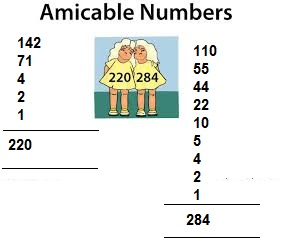# Learn Amicable Numbers with Example#Definition Of Amicable Numbers

An online amicable number definition

Amicable numbers are pairs of numbers, each of which is the sum of the others aliquot divisors.Example, 220 and 284 are amicable numbers whereas all the aliquot divisors of 220, i.e., 110, 55, 44, 22, 10, 5, 4, 2, 1 add up to 284 and all the aliquot divisors of 284, i.e., 142, 71, 4, 2, 1 add up to 220.

Also true for any two amicable numbers, N1 and N2, is the fact that the sum of all the factors/divisors of both, Sf(N1 + N2) = N1 + N2. Stated another way, Sf(220 + 284) = 220 + 284 = 504.#Related Number Types

##Math number calculation ►

Online Algebra calculation, formulas , Digital calculation, Statistical calculation, Math Converters Pet Age Calculator